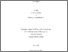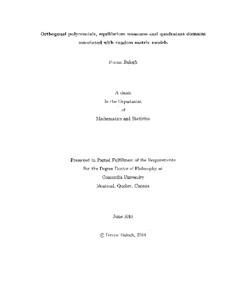Title:

# Orthogonal polynomials, equilibrium measures and quadrature domains associated with random matrix models

Balogh, Ferenc (2010) Orthogonal polynomials, equilibrium measures and quadrature domains associated with random matrix models. PhD thesis, Concordia University.Preview Text (application/pdf) NR71148.pdf - Accepted Version 8MB

## Abstract

Motivated by asymptotic questions related to the spectral theory of complex random matrices, this work focuses on the asymptotic analysis of orthogonal polynomials with respect to quasi-harmonic potentials in the complex plane. The ultimate goal is to develop new techniques to obtain strong asymptotics (asymptotic expansions valid uniformly on compact subsets) for planar orthogonal polynomials and use these results to understand the limiting behavior of spectral statistics of matrix models as their size goes to infinity. For orthogonal polynomials on the real line the powerful Riemann-Hilbert approach is the main analytic tool to derive asymptotics for the eigenvalue correlations in Hermitian matrix models. As yet, no such method is available to obtain asymptotic information about planar orthogonal polynomials, but some steps in this direction have been taken. The results of this thesis concern the connection between the asymptotic behavior of orthogonal polynomials and the corresponding equilibrium measure. It is conjectured that this connection is established via a quadrature identity: under certain conditions the weak-star limit of the normalized zero counting measure of the orthogonal polynomials is a quadrature measure for the support of the equilibrium measure of the corresponding two-dimensional electrostatic variational problem of the underlying potential. Several results are presented on equilibrium measures, quadrature domains, orthogonal polynomials and their relation to matrix models. In particular, complete strong asymptotics are obtained for the simplest nontrivial quasi-harmonic potential by a contour integral reduction method and the Riemann-Hilbert approach, which confirms the above conjecture for this special case

Divisions: Concordia University > Faculty of Arts and Science > Mathematics and Statistics Thesis (PhD) Balogh, Ferenc xiii, 285 leaves : ill. ; 29 cm. Concordia University Ph. D. Mathematics 2010 Harnad, John LE 3 C66M38P 2010 B35 979424 Concordia University Library 09 Dec 2014 17:59 13 Jul 2020 20:12 https://concordiauniversity.on.worldcat....
All items in Spectrum are protected by copyright, with all rights reserved. The use of items is governed by Spectrum's terms of access.

Repository Staff Only: item control pageResearch related to the current document (at the CORE website)
Back to top